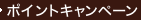•### Fixed Point Theorems with Applications to Economics and Game Theory （REP SUB）

• 提携先の海外書籍取次会社に在庫がございます。通常2週間で発送いたします。
重要ご説明事項
1. 納期遅延や、ご入手不能となる場合が若干ございます。
2. 複数冊ご注文の場合、分割発送となる場合がございます。
3. 美品のご指定は承りかねます。
• ≪洋書のご注文につきまして≫ 「海外取次在庫あり」および「国内仕入れ先からお取り寄せいたします」表示の商品でも、納期の目安期間内にお届けできないことがございます。あらかじめご了承ください。

• 製本 Paperback:紙装版/ペーパーバック版
• 言語 ENG
• 商品コード 9780521388085
• DDC分類 330

### Full Description

One of the problems in economics that economists have devoted a considerable amount of attention in prevalent years has been to ensure consistency in the models they employ. Assuming markets to be generally in some state of equilibrium, it is asked under what circumstances such equilibrium is possible. The fundamental mathematical tools used to address this concern are fixed point theorems: the conditions under which sets of assumptions have a solution. This book gives the reader access to the mathematical techniques involved and goes on to apply fixed point theorems to proving the existence of equilibria for economics and for co-operative and noncooperative games. Special emphasis is given to economics and games in cases where the preferences of agents may not be transitive. The author presents topical proofs of old results in order to further clarify the results. He also proposes fresh results, notably in the last chapter, that refer to the core of a game without transitivity. This book will be useful as a text or reference work for mathematical economists and graduate and advanced undergraduate students.

### Table of Contents

`Preface                                            vii  Introduction: models and mathematics             1  (8)  Convexity                                        9  (10)  Simplexes                                        19 (4)  Sperner's lemma                                  23 (3)  The Knaster-Kuratowski-Mazurkiewicz lemma        26 (2)  Brouwer's fixed point theorem                    28 (3)  Maximization of binary relations                 31 (7)  Variational inequalities, price equilibrium,     38 (6)  and complementarity  Some interconnections                            44 (6)  What good is a completely labeled subsimplex     50 (3)  Continuity of correspondences                    53 (10)  The maximum theorem                              63 (4)  Approximation of correspondences                 67 (2)  Selection theorems for correspondences           69 (2)  Fixed point theorems for correspondences         71 (3)  Sets with convex sections and a minimax          74 (4)  theorem  The Fan-Browder theorem                          78 (3)  Equilibrium of excess demand correspondences     81 (7)  Nash equilibrium of games and abstract           88 (7)  economies  Walrasian equilibrium of an economy              95 (9)  More interconnections                            104(5)  The Knaster-Kuratowski-Mazurkiewicz-Shapley      109(3)  lemma  Cooperative equilibria of games                  112(10)References                                         122(5)Index                                              127`